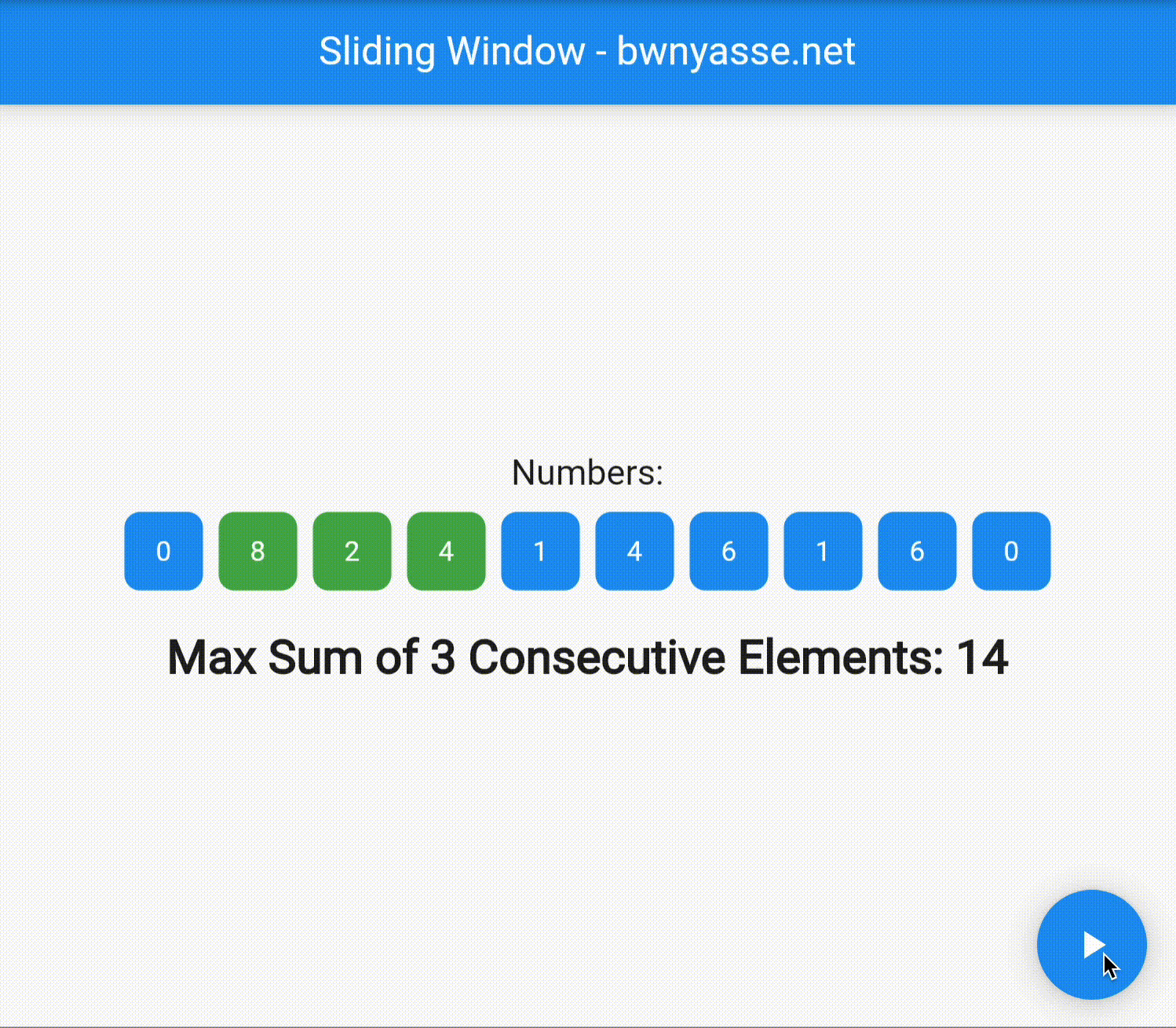# Design Pattern: The Sliding Window in DartWelcome to the “Design Pattern of the Month” series! In this monthly blog series, we will explore various design patterns and their implementation in Dart using Flutter. Each month, we will dive deep into a specific design pattern, providing detailed explanations and practical implementations. To kick off the series, let’s explore the Sliding Window pattern and its implementation in Dart using Flutter. We will build a Flutter application to visualize the pattern in action.## 1 - What is the Sliding Window Pattern?

The Sliding Window pattern is a powerful technique used in algorithmic problem-solving to efficiently solve problems involving arrays or strings. It involves maintaining a set of elements within a “window” of fixed or variable size and sliding the window through the given data structure. By doing so, we can process the data in a single pass, avoiding redundant computations and achieving better time complexity.

### Common Use Cases:

The Sliding Window pattern is particularly useful in solving a variety of problems, including but not limited to:

• Finding the maximum or minimum subarray/substring of a given size.
• Calculating the sum or average of all subarrays/substrings of a given size.
• Detecting patterns or subsequences within an array or string.
• Solving problems involving intervals or time windows.

## 2 - Implementation in Dart with Flutter:

To demonstrate the Sliding Window pattern in action, we will build a Flutter application that showcases the Maximum Sum Subarray problem. This problem involves finding the subarray of a given size with the maximum sum. Let’s take a look at the implementation steps:

### Step 1: Setting up the Flutter Application

• Set up a new Flutter project using the flutter `create command`.
• Open the project in your preferred IDE or editor.

### Step 2: Creating the SlidingWindowScreen Widget

• Create a new Dart file, `sliding_window_screen.dart`, and define a `SlidingWindowScreen` widget that extends `StatefulWidget`.
• Inside the `SlidingWindowScreen` widget, declare the necessary variables like the list of numbers and the window size.
• Implement the build method to display the list of numbers and the maximum sum subarray.

### Step 3: Implementing the Sliding Window Algorithm

• Within the `SlidingWindowScreen` widget, create a method, let’s name it `findMaxSumSubarray`, to implement the Sliding Window algorithm.
• Initialize variables for the current sum, maximum sum, and the starting index of the subarray.
• Iterate through the list of numbers, adjusting the window boundaries and updating the sums accordingly.
• Track the starting index of the maximum sum subarray.
• Finally, update the UI to display the maximum sum subarray.

### Step 4: Displaying the Visuals

• Customize the UI to visually represent the list of numbers, highlighting the maximum sum subarray.
• You can use Container widgets with different colors to represent the numbers, highlighting the subarray using a specific color.

### Step 5: Running the Application

• Run the Flutter application on a device or emulator to see the Sliding Window pattern in action.
• Observe how the maximum sum subarray updates as the window slides through the list of numbers.

## 3 - Implementation of the findMaxSum Function

The heart of the Sliding Window pattern lies in the implementation of the `findMaxSum` function. This function efficiently finds the maximum sum of a subarray of a given size within a list of numbers using the sliding window technique. Let’s dive deeper into how this function works:

findMaxSum
`````` 1int findMaxSum() {
2  int maxSum = 0;
3  int currentSum = 0;
4  int maxSumIndex = 0;
5
6  // Calculate sum of first 'k' elements
7  for (int i = 0; i < k; i++) {
8    currentSum += numbers[i];
9  }
10
11  maxSum = currentSum;
12
13  // Slide the window by one element at a time
14  for (int i = k; i < numbers.length; i++) {
15    currentSum = currentSum - numbers[i - k] + numbers[i];
16    if (currentSum > maxSum) {
17      maxSum = currentSum;
18      maxSumIndex = i - k + 1;
19    }
20  }
21
22  maxSumElements = List<int>.generate(k, (index) => maxSumIndex + index);
23
24  return maxSum;
25}``````

The `findMaxSum` function utilizes two pointers, `currentSum` and `maxSum`, to keep track of the current sum and the maximum sum encountered so far. It also maintains the `maxSumIndex` variable, which represents the starting index of the subarray with the maximum sum.

To initialize the window, we calculate the sum of the first `k` elements using a simple for loop. This sets the initial values of `currentSum` and `maxSum`.

Next, we slide the window by one element at a time. Starting from the `k` th element, we subtract the value of the element that falls outside the window (`numbers[i - k]`) and add the value of the new element that enters the window (`numbers[i]`). This sliding operation efficiently updates the `currentSum` without recomputing the sum of the entire subarray.

We then compare the `currentSum` with the `maxSum`. If the `currentSum` is greater, we update the `maxSum` and the `maxSumIndex` accordingly to store the new maximum sum and its starting index.

Finally, we generate the `maxSumElement` list, which contains the indices of the consecutive elements that have the maximum sum. This list allows us to visually highlight the maximum sum subarray in our Flutter application.

The `findMaxSum` function returns the `maxSum`, representing the maximum sum of a subarray of size `k` within the given list of numbers.

Understanding the inner workings of the `findMaxSum` function provides a solid grasp of the Sliding Window pattern’s efficiency and power in solving problems involving subarrays.